Request a call back

# CBSE Class 10 Mathematics Previous Year Question Paper 2020 Delhi Set - 3

Does the sound of Class 10 Maths intrigue you? Or, does it scare you away? All of these happen because it's not easy to handle the Board pressure for CBSE Class 10. But, we will surely help you to tackle the syllabus for Class 10 Maths by providing you with the Class 10 Revision NotesClass 10 Textbook SolutionsClass 10 Tests for all of the chapters available in CBSE Class 10 Maths. We know that scoring more marks in CBSE Class 10 Maths has never been this easy before. But, by referring to all of the study materials we provide at TopperLearning, you can easily score more marks in the Class 10 board examination.

The study materials are created by our subject experts that we offer for CBSE Class 10, very well know the syllabus and essential facts of CBSE Class 10. These study materials will help you understand all the CBSE Class 10 Maths concepts as we focus on providing solutions that simplify a subject's complex fundamentals. At TopperLearning, we believe in delivering quality solutions at a low cost, and we strictly follow the latest CBSE Class 10 syllabus. We make sure that these study materials are revised from time to time. Our TopperLearning packages involve all the study resources for CBSE Class 10, such as Solved question papersvideo lessons and revision notes to help you score high marks. We also provide you with the updated NCERT textbook Solutions and RD Sharma textbook solutions, which provide students with step-by-step explanations.

Our study materials have also introduced the Case Study Based Questions for CBSE Class 10 of all the chapters available in Class 10. These questions are designed based on the latest syllabus of CBSE Class 10.

So why wait when you can quickly get the CBSE class 10 plans.

Question numbers 1 to 10 are multiple choice questions of 1 mark each.

Select the correct option.

Q 1. The point P on x-axis equidistant from the points A(–1, 0) and B(5, 0) is

A. (2, 0)

B. (0, 2)

C. (3, 0)

D. (2, 2)

Q 2. The co-ordinates of the point which is reflection of point (–3, 5) in x-axis are

A. (3, 5)

B. (3, -5)

C. (-3, -5)

D. (-3, 5)

Q 3. If the point P(6, 2) divides the line segment joining A(6, 5) and B(4, y) in the ratio 3:1, then the value of y is

A. 4

B. 3

C. 2

D. 1

Q 4. The sum of exponents of prime factors in the prime-factorisation of 196 is

A. 3

B. 4

C. 5

D. 2

Q 5. Euclid’s division Lemma states that for two positive integers a and b, there exists unique integer q and r satisfying a = bq + r, and

A. 0 < r < b

B. 0 < r < b

C. 0 ≤ r < b

D. 0 ≤ r ≤ b

Q 6. The zeroes of the polynomial x2 – 3x – m(m+3) are

A. m, m+3

B. –m, m+3

C. m, –(m+3)

D. –m, –(m+3)

Q 7. The value of k for which the system of linear equations x + 2y = 3, 5x + ky + 7 = 0 is inconsistent is

A.B.C. 5

D. 10

Q 8. The roots of the quadratic equation x2 – 0.04 = 0 are

A. ±0.2

B. ±0.02

C. 0.4

D. 2

Q 9. The common difference of the A.P.A. 1

B.C. -1

D. 2

Q 10. The nth term of the A.P. a, 3a, 5a, …. Is

A. na

B. (2n – 1)a

C. (2n + 1)a

D. 2na

In Q. Nos. 11 to 15, fill in the blanks. Each question carries 1 mark:

Q 11. In fig. 1, the angles of depressions from the observing positions O1 and O2 respectively of the object A are ______, ______.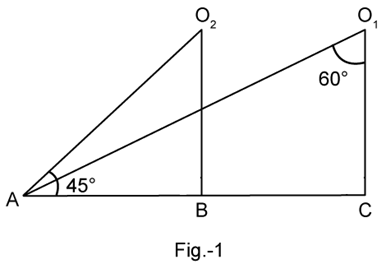Q 12. In ΔABC, AB=6  cm, AC = 12 cm and BC = 6 cm, then ∠B=______.

OR

Two triangles are similar if their corresponding sides are ______.

Q 13.  In given Fig. 2, the length PB=______cm.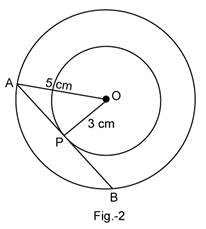Q 14. In fig. 3, MN || BC and AM : MB =1 : 2, then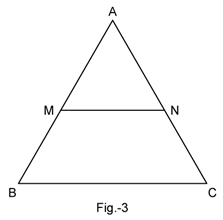Q 15. The value of sin 32° cos 58° +cos 32° sin 58° is ______.

OR

The value ofis ______.

Q Nos. 16 to 20 are short answer type questions of 1 mark each.

Q 16. A die is thrown once. What is the probability of getting a prime number?

Q 17. If a number x is chosen at random from the numbers –3, –2, –1, 0, 1, 2, 3, then find the probability of x< 4.

OR

What is the probability that a randomly taken leap year has 52 Sundays?

Q 18. If sin A + sin2 A = 1, then find the value of the expression (cos2 A + cos4 A).

Q 19. Find the area of the sector of a circle of radius 6 cm whose central angle is 30°. (Take π =3.14).

Q 20. Find the class marks of the classes 20 – 50 and 35 – 60.

Q.Nos.21 to 26 carry 2 marks each.

Q 21. A teacher asked 10 of his students to write a polynomial in one variable on a paper and then to handover the paper.

The following were the answers given by the students:

2x + 3, 3x2 + 7x + 2, 4x3 + 3x2 + 2, x3+ 7, 7x +, 5x3 – 7x + 2, 2x2 + 3 –, 5x –, ax+ bx2 + cx + d, x +.

1. How many of the above ten, are not polynomials?
2. How many of the above ten, are quadratic polynomials?

Q 22 .A child has a die whose six faces show the letters as shown below: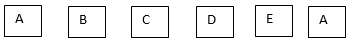The die is thrown once. What is the probability of getting (i) A, (ii) D?

Q 23. In fig. 4, ABC and DBC are two triangles on the same base BC. If AD intersects BC at O, show that.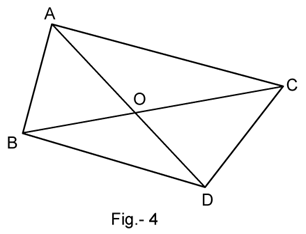OR

In fig.5, if AD ⊥ BC, then prove that AB2 + CD2 = BD2 + AC2.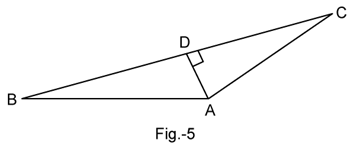Q 24. Prove thatOR

Show that tan4θ + tan2θ = sec4θ - sec2θ

Q 25. Find the mode of the following frequency distribution: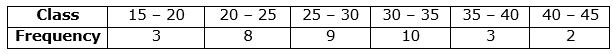Q 26. From a solid right circular cylinder of height 14 cm and base radius 6 cm, a right circular cone of same height and same base radius is removed. Find the volume of the remaining solid.

Q. Nos. 27 to 34 carry 3 marks each.

Q 27. If a circle touches the side BC of a triangle ABC at P and extended sides AB and AC at Q and R, respectively, prove that.

Q 28. The area of a circular play ground is 22176 cm2. Find the cost of fencing this ground at the rate of Rs. 50 per metre.

Q 29. If the mid – point of the line segment joining the points A (3, 4) and B (k, 6) is P(x, y) and x + y – 10 = 0, find the value of k.

OR

Find the area of triangle ABC with A (1, –4) and the mid-points of sides through A being (2, –1) and (0, –1).

Q 30. If Fig.6, if ΔABC ~ ΔDEF and their sides of lengths (in cm) are marked along them, then find the lengths of sides of each triangle.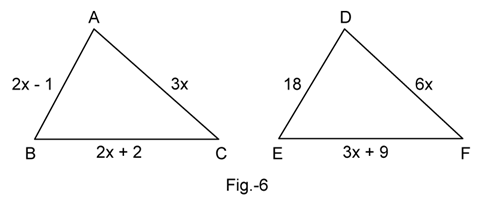Q 31. If 2x + y = 23 and 4x – y = 19, find the value of (5y – 2x) and.

OR

Solve forQ 32. Which term of A.P. 20, 19, 18, 17,....  is the first negative term.

OR

Find the middle term of the A.P. 7, 13, 19, …., 247.

Q 33. Water in a canal, 6m wide and 1.5m deep, is flowing with a speed of 10 km/h. How much area will it irrigate in 30 minutes, if 8cm standing water is required?

Q 34. Show that:Q. Nos. 35 to 40 carry 4 marks each.

Q 35. The mean of the following frequency distribution is 18. The frequency f in the class interval 19 – 21 is missing. Determine f.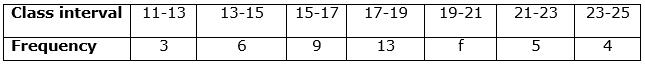OR

The following table gives production yield per hectare of wheat of 100 farms of a village: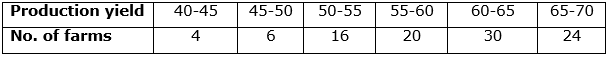Change the distribution to a ‘more than’ type distribution and draw its ogive.

Q 36. From a point on the ground, the angles of elevation of the bottom and the top of a tower fixed at the top of a 20m high building are 45° and 60° respectively. Find the height of the tower.

Q 38. Prove thatis an irrational number.

Q 39. Draw a circle of radius 3.5cm. From a point P, 6cm from its centre, draw two tangents to the circle.

OR

Construct a ΔABC with AB=6cm, BC=5cm and ∠B=60°. Now construct another triangle whose sides aretimes the corresponding sides of ΔABC.

Q 40. A solid is in the shape of hemisphere surmounted by a cone. If the radius of hemisphere and base radius of cone is 7cm and height of cone is 3.5cm, find the volume of the solid. (Take π =).